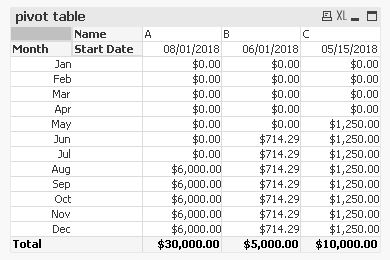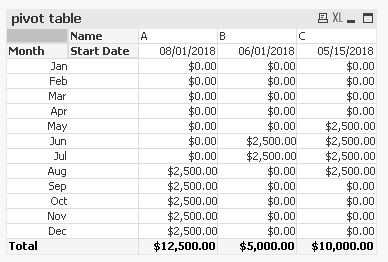# QlikView Scripting

Discussion Board for collaboration on QlikView Scripting.

Highlighted
New Contributor II

## If condition is incorrect in script - problem adding x amount of months to a date

Hello Qlik Community,

I have the following script that divides a yearly labor cost evenly into months based on a starting date. The script works great as it assumes the yearly cost should be split from month([Start Date]) through the end of the year. In other words, monthly cost = yearlyCost / (13 - month([Start Date])). I have several cases where the length of the contract does not continue through the end of the year. There is another field in the data that is the length of the contract which can be used to solve this problem. Below is the code of the working script. I am using Qlik Sense in the cloud.

```table1:
Date([Start Date]) as [Start Date],
Money(yearlyCost) as [yearlyCost],
Month(Date#(IterNo(),'M')) as Month,
Money(If(IterNo()>=Month([Start Date]),[yearlyCost]/(13-Month([Start Date])),0)) as [Direct Labor]
FROM mytable.xlsx (ooxml, embedded labels, table is Sheet1)  While IterNo()<=12;

```

I have changed my if statement in line 6 to the following, which utilizes the field numMonths.

Money(If(IterNo()>=Month([Start Date]) and '\$(Month)' <= Month([StartDate]) + Month(Date#([numMonths], 'M')),[2019 NTC Direct Labor]/numMonths,0)) as [Direct Labor]

This is what the output should look like below except for in the case of name = b, the numMonths = 2 so only June and July should split the total cost at \$2500 each. Same with name = c, spread out over 4 months (May, June, July, August).Any help on getting this working correctly?

Thanks,

Zach

Tags (3)
3 Replies
Honored Contributor III

## Re: If condition is incorrect in script - problem adding x amount of months to a date

pl attach sample excel with expected output

New Contributor II

## Re: If condition is incorrect in script - problem adding x amount of months to a date

I have updated my question. Also, this is the link to the original question, maybe it provides more details.

Load in for each loop that iterates over months

Thanks,

ZachMVP

## Re: If condition is incorrect in script - problem adding x amount of months to a date

Hi,

one possible solution using your sample data:```table1:
Date([Start Date]) as [Start Date],
Money([Yearly Direct Labor]) as [Yearly Direct Labor],
Month(Date#(IterNo(),'M')) as Month,
numMonths,
Money(If(IterNo()>=Month([Start Date]) and IterNo()<Month([Start Date])+numMonths,[Yearly Direct Labor]/numMonths,0)) as [Direct Labor]